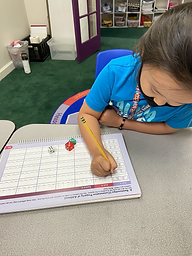Ms. Joan

Target 1​

Lesson Type:

Review

Number Operation

:

Operational Relationships

Use properties of operations as strategies for adding and subtracting.

1:

Identify the Commutative Property of Addition.

2:

Understand that changing the order of addends, does not change the sum.

3:

Understand the inverse relationship between addition and subtraction.

Kindergarden

Vocabulary:

Activities:

Student were given dominoes. Then, wrote the number on the paper and identified that even when the numbers changed their position, they will have the same sum.

• Student was asked to roll the dice, put it in a number sentence and solve it. Despite the different ways to show adding 3 different numbers, we will still have the same sum.
• Student were given 3 numbers that is part of the fact families. We use this numbers to show its relationship when adding and subtracting.
• Student made a sample of fact family house. Rolled dice, find out the answer and show how it these 3 numbers may be related in adding and subtracting.Home Exploration

Guiding Questions:Absent Students:

Target 2

:

Vocabulary:

Activities:Home Exploration

Guiding Questions:Target 3

:

Vocabulary:

Activities:Home Exploration